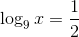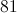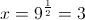# PSAT Math : How to find an exponent from a rational number

## Example Questions

### Example Question #1 : How To Find An Exponent From A Rational Number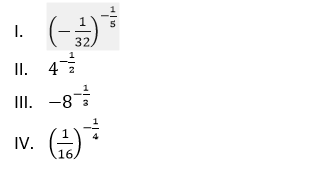Which of the following lists the above quantities from least to greatest?

I, IV, II, III

I, IV, III, II

IV, III, II, I

II, III, I, IV

I, III, II, IV

I, III, II, IV

Explanation: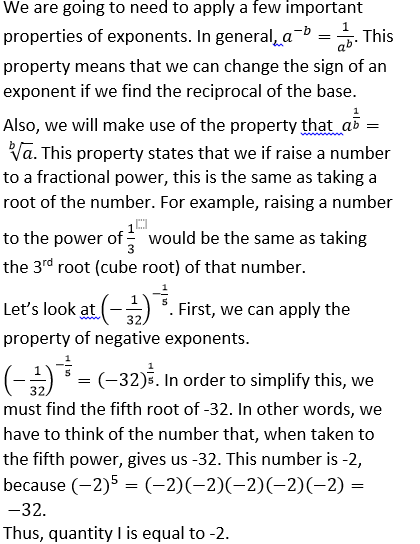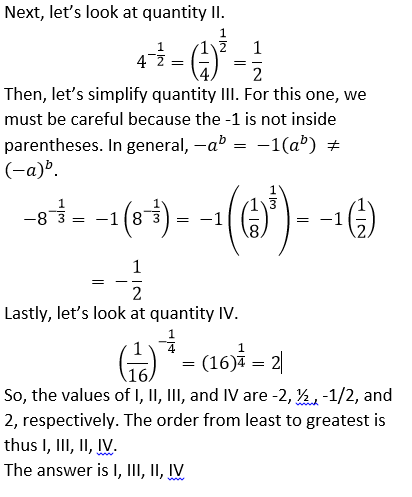### Example Question #2 : How To Find An Exponent From A Rational Number

Solve for.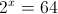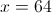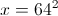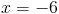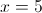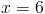Explanation:

Since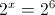Hence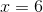### Example Question #3 : How To Find An Exponent From A Rational Number

Simplify: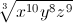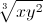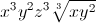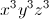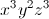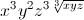Explanation: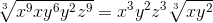### Example Question #4 : How To Find An Exponent From A Rational Number

Solve for: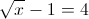Explanation:

From the equation one can see that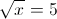Hencemust be equal to 25.

### Example Question #5 : How To Find An Exponent From A Rational Number

Evaluate: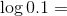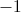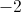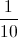Explanation: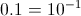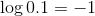### Example Question #6 : How To Find An Exponent From A Rational Number

Solve for.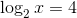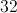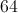Explanation: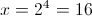### Example Question #7 : How To Find An Exponent From A Rational Number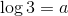and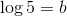Find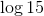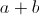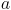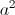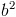Explanation: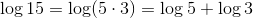Hence the correct answer is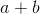.

### Example Question #8 : How To Find An Exponent From A Rational Number

Solve for.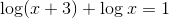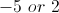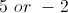Explanation:

Use the rules of logarithms to combine terms.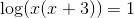Hence,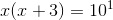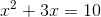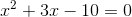By fatoring we get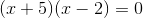Hence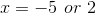.

However, you cannot take the logarithm of a negative number. Thus, the only value foris.

### Example Question #9 : How To Find An Exponent From A Rational Number

If a piece of pie is cut into 3 sections, and each of those pieces is further cut into three sections, then those pieces are cut into three sections, how many (tiny) pieces of pie are there?

36

125

12

27

40

27

Explanation:

The answer is 33 = 27

### Example Question #10 : How To Find An Exponent From A Rational Number

Solve for.